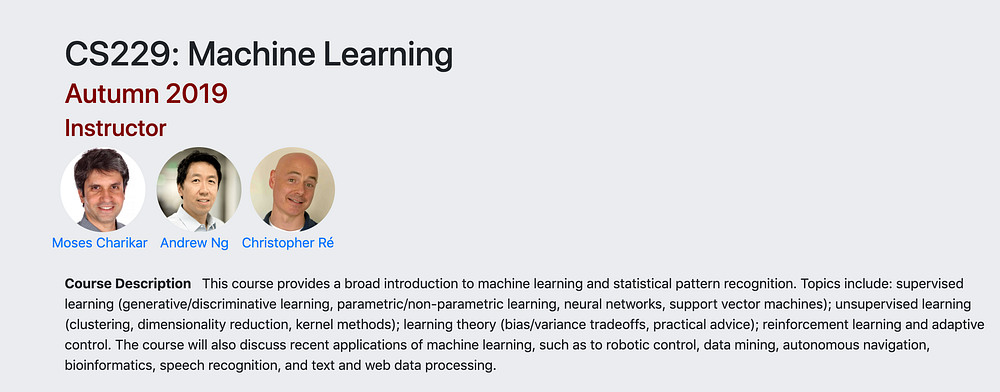# Summary of algorithms in Stanford Machine Learning (CS229) Part I

Source: Deep Learning on Medium## Supervised learning in general and notations

The key point is that supervised learning has both feature x and label y (while unsupervised learning only has feature x but not label). Supervised learning has a set of training examples (x, y) and the goal is to learn a hypothesis h that can map x to y. In order to make predictions, we give a new x’ that is not in the training set and use h learned above to predict y. So y_prediction can be written as h(x’).

x is usually a vector of n features, denoted as x subscript i as: {x_{1}, x_{2}, …, x_{n}}

y is a constant

We use superscript i to denote ith training example. For instance x^{1} represents the feature vector of first training example.

There are two types of supervised learning. If our prediction is continuous such as housing price, sales estimation, this is called regression problem. If our prediction is discrete, such as spam or non-spam email, like or don’t like, this is called classification problem.

## Linear regression

This is one of the most widely used regression algorithms. The assumption is that our hypothesis h has a linear relationship with x defined as follows: